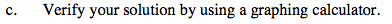### Home > PC > Chapter 2 > Lesson 2.1.2 > Problem2-23

2-23.
1. Sketch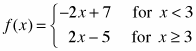. 2-23 HW eTool (Desmos) Homework Help ✎

1. This graph should look like a function you have seen before, that is, the absolute value function. Write f (x) using the absolute value function with the necessary transformations.

2. Shift f(x) five units to the left and three units down. Write the new function, g(x), as an absolute value function.

3. Verify your solution by using a graphing calculator.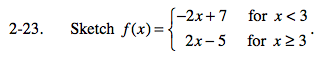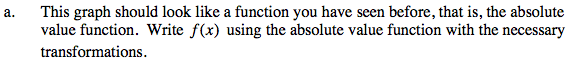Graph two lines. Sketch the first one to the right of x = 3, the second one to the left of x = 3.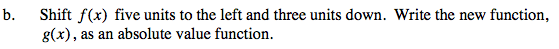This is f(x + 5) − 3.
Change the domain for the horizontal shift only.